神经网络编码分类变量—categorical_embedder

1.神经网络的数据预处理

​ 机器学习模型离不开数据的预处理，预处理对于构建网络模型同样非常重要往往能决定训练结果，对于不同的数据集，预处理方法都会有或多或少的局限性和特殊性，特别是神经网络输入数据仅支持浮点数张量。无论处理什么数据（声音，图像还是文本），都需要将其转化为张量，然后再提供给神经网络模型。当我们遇到比如这些数据可以通过序号编码（Ordinal Encoding）、独热编码（One-hot Encoding）、二进制编码 （Binary Encoding）等方法将其转化为数字。接下来介绍一种更为先进的神经网络编码方法。

2.什么是embedding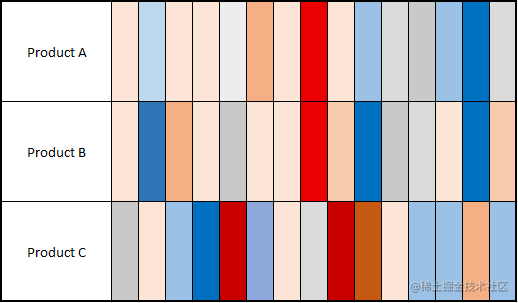​ 每个类别都映射到一个不同的向量，并且在训练神经网络时可以调整或学习向量的属性。向量空间提供类别的投影，从而使得那些接近或相关的类别自然地聚在一起。为了学习嵌入，我们创建了一个使用这些嵌入作为特征并与其他特征交互以学习上述任务。此时需要引用一个概念：词向量。

2.1 词向量

​ 词向量是一种语言的每个词的嵌入向量。词向量的整个想法是，在句子中出现更近的词通常彼此更接近。嵌入是n 维向量。每个维度捕获每个单词的某些属性/属性，因此更接近属性，更接近单词。为了学习词向量，我们创建了一组出现在一个小词窗口（比如 5 个词）内的词对作为正例，并创建一组没有出现在该窗口中的词对作为负例。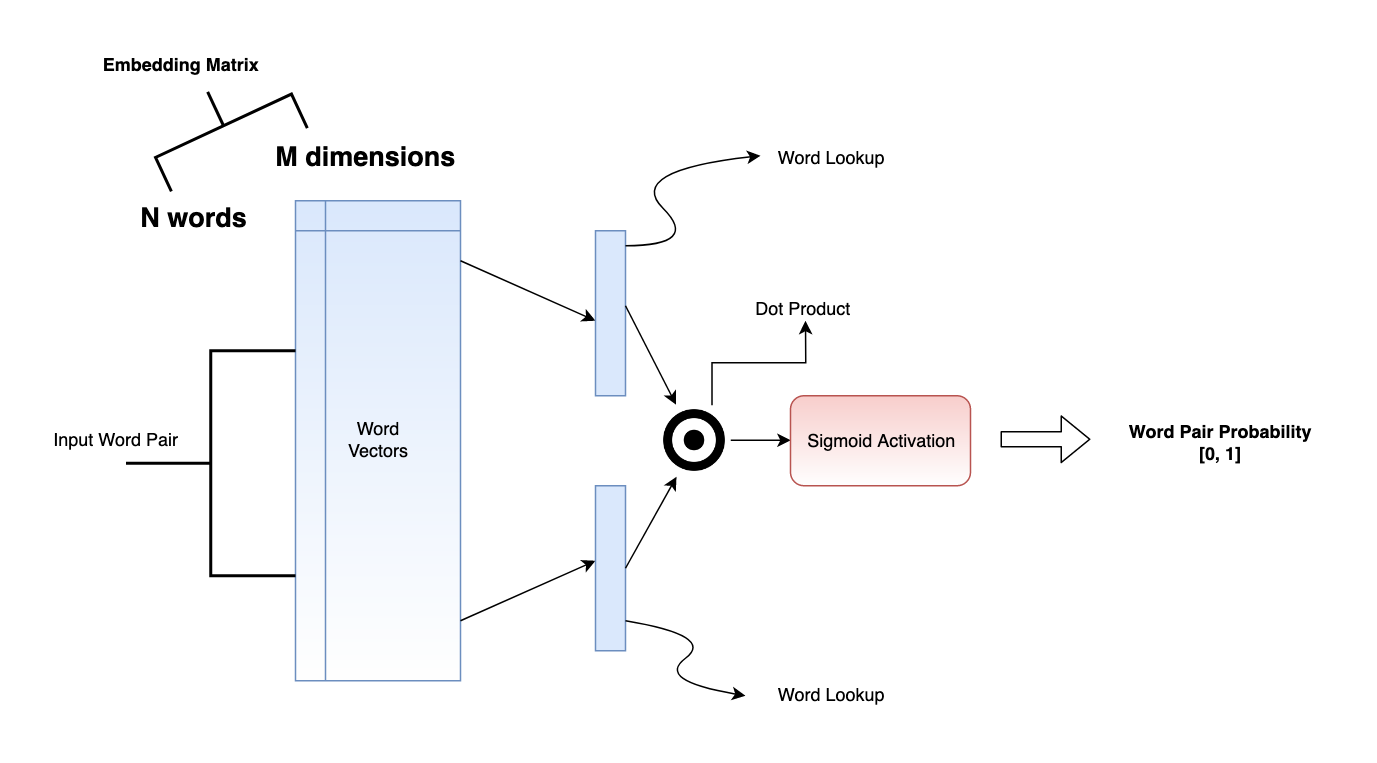​ 当我们在足够大的数据集上训练上述神经网络时，模型会学习预测两个词是否相关。然而，这个模型的副产品是嵌入矩阵，它是词汇表中每个单词的信息丰富的向量表示。(参考Categorical Embedding and Transfer Learning一篇)

2.2传统方法
• 序号编码（Ordinal Encoding）序号编码通常用于处理类别间具有大小关系的数据。
• 独热编码（One-hot Encoding）使用稀疏向量来节省空间，配合特征选择来降低维度
• 二进制编码 （Binary Encoding）二进制编码主要分为两步，先用序号编码给每个类别赋予一个类别ID，然后 将类别ID对应的二进制编码作为结果。
2.3 categorical_embedder工作原理

​ 首先，每个分类变量类别都映射一个n维向量。这种映射是在标准的监督训练过程中由神经网络学习的。如果我们想使用上述15维ID作为特征，那么我们将以监督方式训练神经网络，获取每个特征的向量并生成如下所示的3*15的矩阵。如下图所示（太多神经节点不好表示，下图仅参考）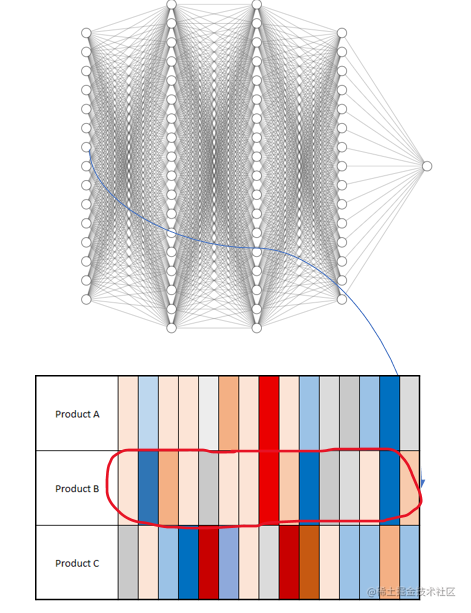​ 接下来，我们将使用数据中对应的向量来替换每个类别。这样做的优点在于我们限制了每个类别所需的列的数量。这在列具有高基数是非常有用（基数是指对集合元素数量的度量）。从神经网络获得的生成嵌入揭示了分类变量的内在属性。这意味着相似的类别将具有相似的嵌入。

2.4 学习嵌入矩阵

​ 嵌入矩阵是浮点数N*M矩阵。这里N是唯一类别的数量，M是嵌入维度。我们决定M的值。通常将M设置为等于N的平方根，然后根据需要增加或减少。实际上，嵌入矩阵是向量的查找表。嵌入矩阵的每一行都是一个唯一类别的向量。

​ 对于公司而言，它有三个产品，每个产品的评价为五万个唯一值，要构建分类嵌入，我们需要解决有意义的任务深度学习模型，在上述任务中使用嵌入矩阵来表示分类变量。我们用15维变量来预测公司的产品关联。可以通过颜色的区分来分析哪个产品具有相关性，当然这个属于推荐系统的一个分析思路。更多的是将样本的属性分门别类构建对应的embedding矩阵，通过这些Label_embedding矩阵，通过Faltten层，输入神经网络中进行训练

3 基于Python的categorical_embedder

3.1 神经网络编码代码复现

cmd pip install categorical_embedder

​ 注意：这个库要求tensorflow的版本在2.1以下，高于此版本会出现未知错误。

​ 在这个categorical_embedder包含一些重要的函数定义，我们仔细描述其含义。

• ce.get_embedding_info(data,categorical_variables=None)：这个函数的目的是识别数据中所有的分类变量，确定其嵌入大小。分类变量的嵌入大小由至少50个或一半的数量决定。唯一值，即嵌入列大小 = Min（50，#该列中的唯一值）。我们可以在categorical_variables参数中传递一个明确的分类变量列表。如果没有，这个函数会自动接受所有数据类型为对象的变量。

```python def get_embedding_info(data, categorical_variables=None): ''' this function identifies categorical variables and its embedding size :data: input data [dataframe] :categorical_variables: list of categorical_variables [default: None] if None, it automatically takes the variables with data type 'object' embedding size of categorical variables are determined by minimum of 50 or half of the no. of its unique values. i.e. embedding size of a column = Min(50, # unique values of that column) ''' if categorical_variables is None: categorical_variables = data.select_dtypes(include='object').columns

return {col:(data[col].nunique(),min(50,(data[col].nunique()+ 1) //2)) for col in categorical_variables}

```

• ce.get_label_encoded_data(data, categorical_variables=None)：此函数标签使用sklearn.preprocessing.LabelEncoder 函数对所有分类变量进行编码（整数编码），并返回标签的数据帧进行训练。Keras/TensorFlow 或任何其他深度学习库都希望数据采用这种格式。

```python def get_label_encoded_data(data, categorical_variables=None): ''' this function label encodes all the categorical variables using sklearn.preprocessing.labelencoder and returns a label encoded dataframe for training :data: input data [dataframe] :categorical_variables: list of categorical_variables [Default: None] if None, it automatically takes the variables with data type 'object' ''' encoders = {}

df = data.copy()

if categorical_variables is None:
categorical_variables = [col for col in df.columns if df[col].dtype == 'object']

for var in categorical_variables:
#print(var)
encoders[var] = __LabelEncoder__()
df.loc[:, var] = encoders[var].fit_transform(df[var])

return df, encoders

```

• ce.get_embeddings(X_train, y_train, categorical_embedding_info=embedding_info, is_classification=True, epochs=100,batch_size=256) ：这个函数训练一个浅层神经网络并返回分类变量的嵌入。在底层是一个二层神经网络架构，具有1000500的神经元，带有ReLU激活。它需要四个必须输出X_train,y_train,categorical_embedding_info：get_embedding_info函数的输出和is_classification:True*用于分类任务；False用于回归任务。

```python def get_embeddings(X_train, y_train, categorical_embedding_info, is_classification, epochs=100, batch_size=256): ''' this function trains a shallow neural networks and returns embeddings of categorical variables :X_train: training data [dataframe] :y_train: target variable :categorical_embedding_info: output of get_embedding_info function [dictionary of categorical variable and it's embedding size] :is_classification: True for classification tasks; False for regression tasks :epochs: num of epochs to train [default:100] :batch_size: batch size to train [default:256] It is a 2 layer neural network architecture with 1000 and 500 neurons with 'ReLU' activation for classification: loss = 'binary_crossentropy'; metrics = 'accuracy' for regression: loss = 'mean_squared_error'; metrics = 'r2' '''

numerical_variables = [x for x in X_train.columns if x not in list(categorical_embedding_info.keys())]

inputs = []
flatten_layers = []

for var, sz in categorical_embedding_info.items():
input_c = Input(shape=(1,), dtype='int32')
embed_c = Embedding(*sz, input_length=1)(input_c)
flatten_c = Flatten()(embed_c)
inputs.append(input_c)
flatten_layers.append(flatten_c)
#print(inputs)

input_num = Input(shape=(len(numerical_variables),), dtype='float32')
flatten_layers.append(input_num)
inputs.append(input_num)

flatten = concatenate(flatten_layers, axis=-1)

fc1 = Dense(1000, kernel_initializer='normal')(flatten)
fc1 = Activation('relu')(fc1)

fc2 = Dense(500, kernel_initializer='normal')(fc1)
fc2 = Activation('relu')(fc2)

if is_classification:
output = Dense(1, activation='sigmoid')(fc2)

else:
output = Dense(1, kernel_initializer='normal')(fc2)

nnet = Model(inputs=inputs, outputs=output)

x_inputs = []
for col in categorical_embedding_info.keys():
x_inputs.append(X_train[col].values)

x_inputs.append(X_train[numerical_variables].values)

if is_classification:
loss = 'binary_crossentropy'
metrics='accuracy'
else:
loss = 'mean_squared_error'
metrics=r2

nnet.fit(x_inputs, y_train.values, batch_size=batch_size, epochs=epochs, validation_split=0.2, callbacks=[TQDMNotebookCallback()], verbose=0)

embs = list(map(lambda x: x.get_weights(), [x for x in nnet.layers if 'Embedding' in str(x)]))
embeddings = {var: emb for var, emb in zip(categorical_embedding_info.keys(), embs)}
return

```

​ 注意这些代码为该库源代码，只需了解即可。

3.2 案例分析—二手车价格预测

​ 基于给定的二手车交易样本数据作为训练，验证，测试样本。构建二手车零售交易价格预测模型。首先要查看整个数据的结构，指标的意义。通过观察数据我们可以发现有一些指标是日期，未知特征等非结构化数据。如果我们想构建机器学习模型(神经网络模型)。

​ 我们需要先使用categorical_embedding方法将非结构数据进行转化，再进行处理。首先导入相关库；

python import pandas as pd import numpy as np import categorical_embedder as ce from sklearn.model_selection import train_test_split from keras import models from keras import layers import matplotlib.pyplot as plt import csv

​ 观察整个数据集的形状并输出前五行观察数据集的内容，部分展示如下；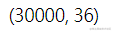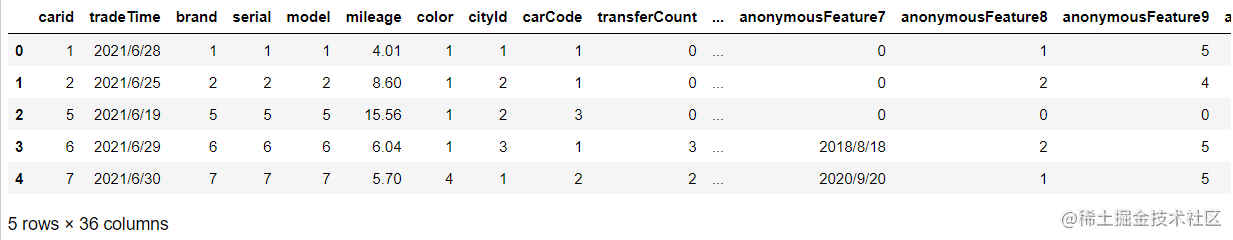python X = train_data.drop(['carid', 'price'], axis=1) y = train_data['carid'] embedding_info = ce.get_embedding_info(X) embedding_info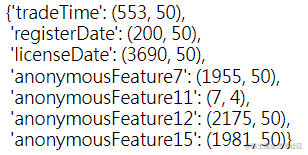​ 我们可以看到需要编码训练的数据部分，接下来需要使用ce.get_label_encoded_data：此函数标签使用sklearn.preprocessing.LabelEncoder对所以分类变量进行编码（整数编码），并返回标签编码的数据帧进行训练。

```python

分割数据

X_train, X_test, y_train, y_test = train_test_split(X_encoded, y)

ce.get_embeddings trains NN, extracts embeddings and return a dictionary containing the embeddings

embeddings = ce.get_embeddings(X_train, y_train, categorical_embedding_info=embedding_info, is_classification=True,epochs=100, batch_size=256) embeddings ```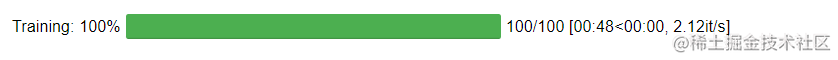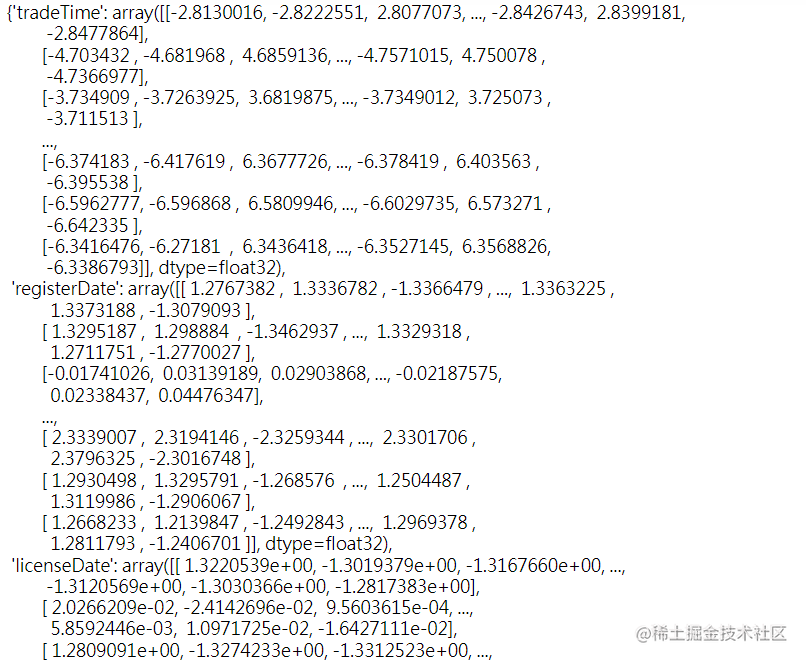​ 如上图所示我们通过一个2层神经网络架构1000*500个神经元，并带有”ReLU激活。我们可以查看一下编码后数据的变化情况，如下图所示；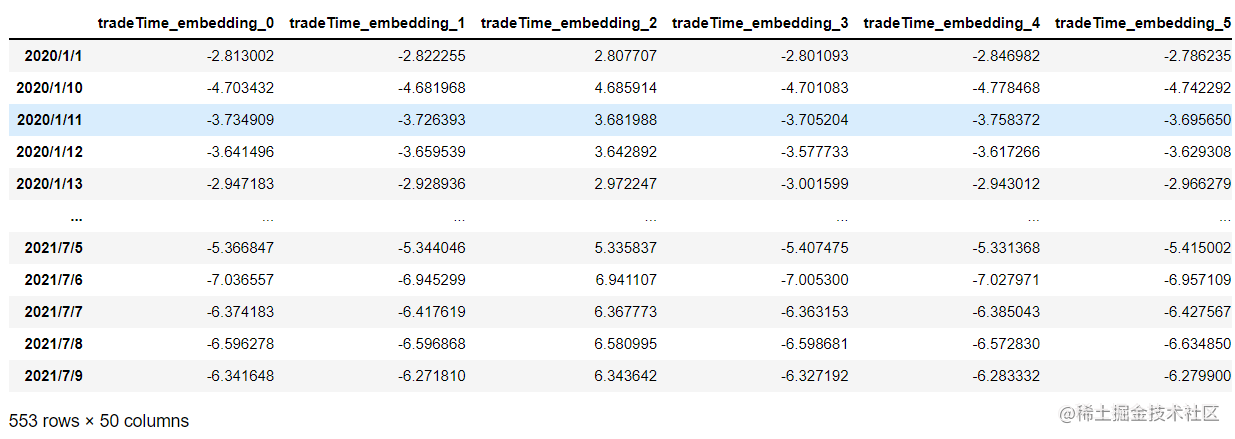​ 接下来需要将训练好的数据编码从字典转换到数据集中；

python data = ce.fit_transform(X, embeddings=embeddings, encoders=encoders, drop_categorical_vars=True) data.head()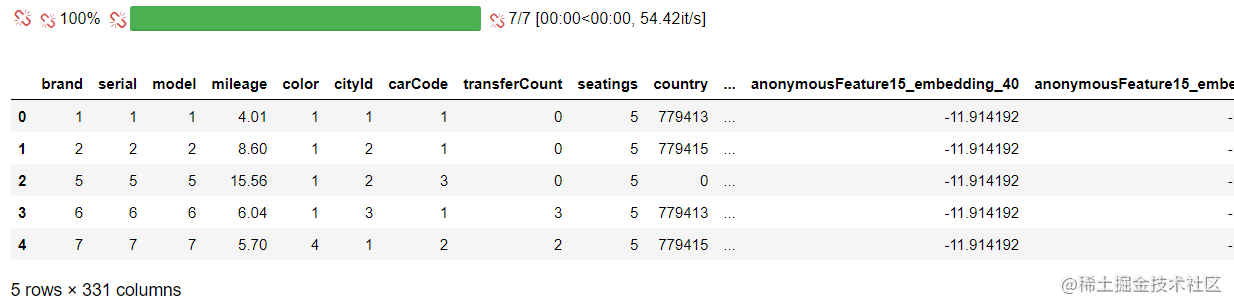​ 此时使用神经网络编码的步骤已经全部结束，从数据头部可以很清晰的看到数据的结构已经全部转换为浮点数张量，已经满足了机器学习模型的输入需求，为了进一步提高模型的效果，还需要进行标准化等方法进行处理。

4 总结

​ 机器学习模型对数字变量比较敏感，对于非结构化数据十分迟钝。传统的分类变量方法一定程度上限制了算法的能力。在适当的条件下，我们可以学习全新的嵌入来提高模型性能。分类嵌入通常表现很好，有助于模型更好的泛化。